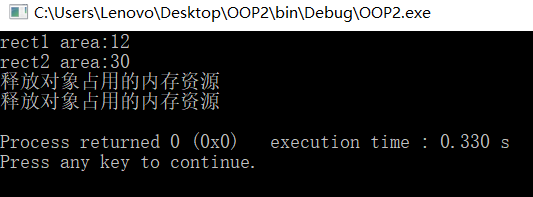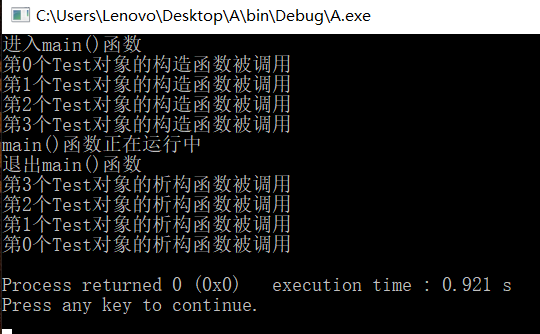# C++ 析构函数

## C++ 析构函数

#### 析构函数例子1

$\qquad$ 析构函数的主要功能是在创建对象时对对象进行初始化操作。与构造函数不同的是，析构函数一般在程序结束前完成对对象的销毁工作。
$\qquad$ 若一个对象中含有指针数据成员，该指针数据成员指向某一个内存块。通常需要在析构函数中将该指针指向的内存块释放，然后销毁对象。
$\qquad$ 如果程序中的内存在使用完后没有得到释放，就很容易造成内存泄露，会导致应用程序运行效率降低，甚至操作系统崩溃，所以要学会利用析构函数释放系统占用的资源。

// 析构函数的使用

#include <iostream>

using namespace std;

class Carea {
int *width, *height;    // 默认为私有变量
public:
Carea(int, int);        // 构造函数的声明
~Carea();               // 析构函数的声明
int area();             // 计算面积的成员函数
};

// 以下为构造函数的定义
Carea::Carea(int a, int b){
width = new int;        // 定义一个整形指针变量width
height = new int;       // 定义一个整形指针变量height
*width = a;             // 把参数a的值赋给指针变量width
*height = b;            // 把参数b的值赋给指针变量height
}

// 以下为析构函数的定义
Carea::~Carea(){
delete width;           // 释放指针变量width占用的内存资源
delete height;          // 释放指针变量height占用的内存资源
cout << "释放对象占用的内存资源" << endl;  // 调用析构函数的信息提示
}

int Carea::area(){          // 计算面积的成员函数的实现
return ((*width)*(*height));    // 通过函数值返回面积
}

int main(){
Carea rect1(3, 4), rect2(5, 6);
cout << "rect1 area:" << rect1.area() << endl;
cout << "rect2 area:" << rect2.area() << endl;
return 0;
}#### 析构函数例子2–调用次序

#include <iostream>

using namespace std;

class Test
{
private:
int num;
public:
Test(int a)
{
num = a;
cout << "第" << num << "个Test对象的构造函数被调用" << endl;
}
~Test()
{
cout << "第" << num << "个Test对象的析构函数被调用" << endl;
}
};

int main()
{
cout << "进入main()函数" << endl;
Test t = {0, 1, 2, 3};       //  定义4个对象，分别以0，1，2，3赋给构造函数的形参a
cout << "main()函数正在运行中" << endl;
cout << "退出main()函数" << endl;
return 0;
}©️2019 CSDN 皮肤主题: 精致技术 设计师: CSDN官方博客